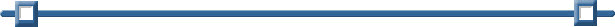MathBits' Activities for the TI-Nspire
(with .tns files) Operating System 2.1

 Algebra 1: • Hitting the Slopes - Students investigate the relationship between slope, y-intercept and linear graphs. The worksheet may be used alone, or in conjunction with the .tns file. Both versions require the use of the TI-Nspire calculator. Hitting the Slopes Intro.pdf Hitting the Slopes Worksheet.pdf HitTheSlope.tns • Examining Linear Equations - Beginning Algebra 1 students examine the algebraic, geometric and graphical ways of investigating the solutions to linear equations. This activity can be used as a class demonstration or as a group activity. A worksheet is included in the document. Algebra tiles are modeled in the geometric section. LinearEquationsAlg1.pdf LinearEquationsAlg1.tns • Fencing the Dog Yard - Students use paper clips to visualize the situation of finding the maximum area of a dog pen with a fixed perimeter. The activity is a two part session. Part I uses the manipulatives to get a feel for the situation and to make conjectures. Part II uses the calculator to do a thorough investigation of the situation. This activity is a good lead in to working with quadratics. Fencing the Dog Yard Intro.pdf Fencing the Dog Yard Lab.pdf Fencing the Dog Yard Calculator.pdf dogyard.tns

 Geometry: • Triangle Inequality Activity - Students follow through a worksheet with their TI-Nspires to discover the Triangle Inequality Theorem. It is assumed that students have a basic familiarity with the use of the calculator.